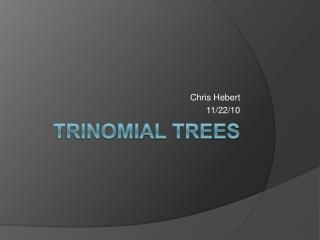DownloadDownload PresentationTrinomial Trees

Trinomial Trees

Télécharger la présentationTrinomial Trees

- - - - - - - - - - - - - - - - - - - - - - - - - - - E N D - - - - - - - - - - - - - - - - - - - - - - - - - - -
Presentation Transcript

1. Chris Hebert 11/22/10 Trinomial Trees

2. History of Trinomial Trees • Developed by Phelim Boyle • 1986 • Binomial Trees (1979)

3. Recall: Binomial Trees • The Binomial Option Pricing Model is essentially a tree that is constructed to show possible values that an underlying asset can take and the resulting value of the option at these values. • The tree is constructed by assuming the stock can only go up or down by a factor related to the length of time and the volatility of the stock.

4. What are Trinomial Trees? • The Trinomial Tree is an adjusted version of the binomial tree. Rather than assuming either up or down, trinomial trees allow for a third option, that the stock price remains constant.

5. Up and down by how much? • Let’s again call the upward movement u, and the downward movement d, while a constant movement will be called m. • The magnitude of this movement is based on volatility and the size of the time interval. • According to Boyle:

6. Boyle vs. CRR • Boyle’s parameters: • Cox-Ross-Rubenstein:

7. Why does Boyle use 2t? • Because we have 3 choices now, we can allow for a bigger change. • Boyle basically combines 2 binomial trees: one were the options are up or even and another where the options are even or down. • Because of the recombining nature of the trees, even-even is the same as up down, so all nodes will fill. • The best way to summarize Boyle’s tree is a binomial tree where we skip every other node.

8. Example • S=90.00 • K=100.0 • T= 1 • σ= .3 • r= .01 • Δt= .1

9. S=90.00 • K=100.0 • T= 1 • σ= .3 • r= .01 • Δt= .1

10. Pull Back Formula • Recall: • We use the exact same formula for the trinomial tree except we take 3 nodes into account. • Also, we use these probabilities for p:

11. Kamrad and Ritchken (1991) • They discovered a different version of the trinomial tree. • They made a few simplifications to the Boyle trinomial tree and prove that it is possible to essentially skip every other step in the binomial tree as a means of generating a trinomial tree.

12. Kamrad and Ritchken (1991) • The derivation of the trinomial tree from the moments of the Brownian motion yields:

13. So How do We Choose λ? • We choose λ so that the option prices given by the tree converge to Black-Scholes as quickly as possible. • Speed is everything for option pricing. • Recall, the drawback for binomial trees is the speed in calculating them. • Trinomial trees allow for a better approximation, with fewer nodes to calculate, so we need less time.

14. What is the Optimal λ? • Kamrad and Ritchken found that the optimal λ is 3/2 • (50,50,.2,.03,1)

15. How does K&R differ from Boyle? • Kamrad and Ritchken streamline the trinomial tree. • They focus the endpoints of the tree on a smaller region of possible end values. • By doing this, they increase speed from the binomial tree, and increase accuracy from Boyle.

16. Recall: Binomial Advantages • Primary advantage of Binomial trees is that American options become much easier to price. • Other advantages • Can factor in dividends • No calculus needed • Easy to make a spreadsheet to find option values

17. Trinomial Trees Advantages • Trinomial trees are calculated in the same way as binomial trees, so all advantages of binomial trees are held intact with trinomial trees.

18. Recall: Binomial Tree Disadvantages/ Limitations • Speed • In order to have accurate results, we want to maximize the number of time periods, but this means creating a lot of nodes which can be slow to calculate even with today’s computers. • For some extreme options, like a cash or nothing, pricing can be inaccurate until a very large number of time intervals is used.

19. Trinomial Tree • Speed is improved from Binomial tree. • We can get more accurate results in fewer intervals, so fewer nodes need to be calculated. • Trinomial trees do a better job of addressing exotic options. • Trinomial trees have more end nodes producing a more accurate layout of payoffs.

20. Call Option: Stock Price: 100, Strike Price: 102, Volatility: 20%, Dividend Yield: 5%, Risk Free: 8%

21. So Why Use Binomial Tree over the Trinomial Tree? • Implementation of the binomial tree is easier. • For vanilla options, the binomial tree converges pretty quickly so the speed advantage and accuracy advantage of the trinomial tree is small.

22. So Why Use the Trinomial Tree over the Binomial Tree? • For exotic options, The trinomial tree can be significantly more accurate and significantly quicker.

23. Works Cited • Albanese, Claudio., and Giuseppe Campolieti. Advanced Derivatives Pricing and Risk Management: Theory, Tools and Hands-on Programming Application. Amsterdam ; Boston: Elsevier Academic Press, 2006. • Berg, Imme van den. Principles of Infinitesimal Stochastic and Financial Analysis: . Singapore ; River Edge, N.J.: World Scientific, 2000. • Daigler, Robert T. Advanced Options Trading: The Analysis and Evaluation of Trading Strategies, Hedging Tactics, and Pricing Models. Chicago, Ill.: Probus Pub. Co., 1994. • http://en.wikipedia.org/wiki/Trinomial_tree • http://www.hoadley.net/options/binomialtree.aspx?tree=T • http://www.global-derivatives.com/index.php/options-database-topmenu/13-options-database/15-european-options#Trinomial • http://www2.warwick.ac.uk/fac/sci/maths/people/staff/oleg_zaboronski/fm/trinomial_tree_2008.pdf • http://bejoymaliakal.com/files/Stochastic%20Calculus%20-%20Binomial%20&%20Trinomial%20Trees.pdf • http://www.haas.berkeley.edu/groups/finance/WP/rpf292.pdf